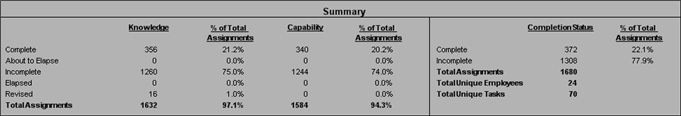# Summaries - Summary Report

The Summary Report actually only has a single summary, and that is the overall summary that appears at the beginning of the report.  The calculations for this summary are the same as for the Qualification Totals Summary.

##• In the far right side of the summary, there is a section named Completion Status.  This could also be known as Combined Status.  This area takes a total of the Tasks and Employees - regardless of which component is present on the Task.

• % of Total Assignments on this section of Complete and Incomplete should always total 100%
Complete (22.1%) + Incomplete (77.9%) = Total (100%)

• Total Assignments = Total Unique Employees X Total Unique Tasks
(1680) = (24) x (70)

• The Complete + About to Elapse = Complete

• The Incomplete + Elapsed + Revised = Incomplete

• The % of Total Assignments for Knowledge and Capability does not always equal 100%, since not all Tasks have a Knowledge Component, and not all Tasks have a Capability Component.  These will only equal 100% if:

• The number of components (Knowledge and Capability) over a total of the Tasks is the same.

• There are only Knowledge components on the Tasks

• There are only Capability components on the Tasks

• The reason for these values are as follows:

• The total number of assignments for either component is the total number of employees assigned to a component X the number of components.

Total Number of Capability Assignments (1584) / number of unique employees (24) = number of tasks with Capability components (66)

Total Number of Knowledge Assignments (1632) / number of unique employees (24) = number of tasks with Knowledge components (68)

Total Assignment of Capability (1584) / Total Assignments (1680) =  Total % Assignment of Capability (94.3)

Total Assignment of Knowledge (1632) / Total Assignments (1680) =  Total % Assignment of Capability (97.1)